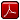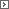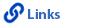Mathematics KindergartenPrintable Version (pdf)Course Introduction

Core Standards of the Course

Strand: MATHEMATICAL PRACTICES (K.MP)
The Standards for Mathematical Practice in Kindergarten describe mathematical habits of mind that teachers should seek to develop in their students. Students will become mathematically proficient in engaging with mathematical content and concepts as they learn, experience, and apply these skills and attitudes (Standards K.MP.1�8).

Standard K.MP.1
Make sense of problems and persevere in solving them. Explain the meaning of a problem, look for entry points to begin work on the problem, and plan and choose a solution pathway. When a solution pathway does not make sense, look for another pathway that does. Explain connections between various solution strategies and representations. Upon finding a solution, look back at the problem to determine whether the solution is reasonable and accurate, often checking answers to problems using a different method or approach.

Standard K.MP.2
Reason abstractly and quantitatively. Make sense of quantities and their relationships in problem situations. Contextualize quantities and operations by using images or stories. Decontextualize a given situation and represent it symbolically. Interpret symbols as having meaning, not just as directions to carry out a procedure. Know and flexibly use different properties of operations, numbers, and geometric objects.

Standard K.MP.3
Construct viable arguments and critique the reasoning of others. Use stated assumptions, definitions, and previously established results to construct arguments. Explain and justify the mathematical reasoning underlying a strategy, solution, or conjecture by using concrete referents such as objects, drawings, diagrams, and actions. Listen to or read the arguments of others, decide whether they make sense, ask useful questions to clarify or improve the arguments, and build on those arguments.

Standard K.MP.4
Model with mathematics. Identify the mathematical elements of a situation and create a mathematical model that shows the relationships among them. Identify important quantities in a contextual situation, use mathematical models to show the relationships of those quantities, analyze the relationships, and draw conclusions. Models may be verbal, contextual, visual, symbolic, or physical.

Standard K.MP.5
Use appropriate tools strategically. Consider the tools that are available when solving a mathematical problem, whether in a real-world or mathematical context. Choose tools that are relevant and useful to the problem at hand, such as physical objects, drawings, diagrams, physical tools, technologies, or mathematical tools such as estimation or a particular strategy or algorithm.

Standard K.MP.6
Attend to precision. Communicate precisely to others by crafting careful explanations that communicate mathematical reasoning by referring specifically to each important mathematical element, describing the relationships among them, and connecting their words clearly to representations. Calculate accurately and efficiently, and use clear and concise notation to record work.

Standard K.MP.7
Look for and make use of structure. Recognize and apply the structures of mathematics, such as patterns, place value, the properties of operations, or the flexibility of numbers. See complicated things as single objects or as being composed of several objects.

Standard K.MP.8
Look for and express regularity in repeated reasoning. Notice repetitions in mathematics when solving multiple related problems. Use observations and reasoning to find shortcuts or generalizations. Evaluate the reasonableness of intermediate results.

Strand: COUNTING AND CARDINALITY (K.CC)
Know number names and the counting sequence (Standards K.CC.1�3). Count to tell the number of objects (Standards K.CC. 4�5). Identify and compare quantities of objects and numerals (Standards K.CC.6�7).Standard K.CC.1.
Count to 100 by ones and by tens.Standard K.CC.2.
Count forward beginning from a given number within the known sequence (instead of having to begin at 1).Standard K.CC.3.
Read and write numbers using base ten numerals from 0 to 20. Represent a number of objects with a written numeral, in or out of sequence (0 represents a count of no objects).Standard K.CC.4.
Understand the relationship between numbers and quantities; connect counting to cardinality.1. When counting objects, say the numbers in the standard order. Pair each quantity of objects with one and only one number and each number with the correct quantity of objects.
2. Understand that the last number said represents the number of objects counted. The number of objects is the same regardless of their arrangement or the order in which they were counted.
3. Understand that each successive number refers to a quantity that is one greater than the previous number.

Standard K.CC.5.
Use counting to answer questions about "how many." For example, 20 or fewer objects arranged in a line, a rectangular array, or circle; 10 or fewer objects in a scattered configuration. Using a number from 1�20, count out that many objects.Standard K.CC.6.
Use matching or counting strategies to identify whether the number of objects in one group is greater than, less than, or equal to the number of objects in another group. Include groups with up to ten objects.Standard K.CC.7
Compare two numbers between 1 and 10 presented as written numerals using "greater than," "less than," or "equal to."Strand: OPERATIONS AND ALGEBRAIC THINKING (K.OA)
Understand addition as putting together and adding to, and understand subtraction as taking apart and taking from (Standards K.OA.1�5).Standard K.OA.1
Represent addition and subtraction with objects, fingers, mental images, simple drawings, or sounds. For example, use clapping, act out situations, and use verbal explanations, expressions, or equations.Standard K.OA.2
Solve addition and subtraction word problems within 10. Use objects or drawings to represent the problem.Standard K.OA.3
Decompose numbers less than or equal to 10 into pairs in more than one way by using objects or drawings. Record each decomposition by a drawing or equation. For example, 5 = 2 + 3 and 5 = 4 + 1.Standard K.OA.4
Make sums of 10 using any number from 1 to 9. For example, 2 + 8 = 10. Use objects or drawings to represent and record the answer.Standard K.OA.5
Fluently add and subtract using numbers within 5.Strand: NUMBER AND OPERATIONS IN BASE TEN (K.NBT)
Compose and decompose numbers 11�19 to gain foundations for place value (Standard K.NBT.1).Standard K.NBT.1
Compose and decompose numbers from 11�19 into ten ones and some further ones. Use objects or drawings and record each composition or decomposition by a drawing or equation. For example, 18 = 10 + 8. Understand that these numbers are composed of ten ones and one, two, three, four, five, six, seven, eight, or nine ones.Strand: MEASUREMENT AND DATA (K.MD)
Describe and compare measurable attributes of objects (Standards K.MD.1�2) and classify objects and count the number of objects in each category (Standard K.MD.3).Standard K.MD.1
Describe measurable attributes of objects, such as length or weight. Describe several measurable attributes of a single object.Standard K.MD.2
Directly compare two objects with a measurable attribute in common, to see which object has "more of"/"less of" the attribute, and describe the difference. For example, directly compare the length of two pencils and describe one as shorter or longer.Standard K.MD.3
Classify objects into given categories; count the numbers of objects in each category and sort the categories by count. Limit the category counts to less than or equal to 10.Strand: GEOMETRY (K.G)
Identify and describe shapes, including squares, circles, triangles, rectangles, hexagons, cubes, cones, cylinders, and spheres (Standards K.G.1�3). Analyze, compare, create, and compose shapes (Standards K.G.4�6).Standard K.G.1
Describe objects in the environment using names of shapes, and describe the relative positions of these objects using terms such as above, below, beside, in front of, behind, and next to.Standard K.G.2
Correctly name shapes regardless of their orientations or overall sizes.Standard K.G.3
Identify shapes as two-dimensional ("flat") or three-dimensional ("solid").Standard K.G.4
Analyze, compare, and sort two- and three-dimensional shapes and objects, in different sizes and orientations, using informal language to describe their similarities, differences, and other attributes (for example, color, size, shape, number of sides).Standard K.G.5
Model and create shapes from components such as sticks and clay balls.Standard K.G.6
Compose simple shapes to form larger shapes. For example, "Can you join these two triangles with full sides touching to make a rectangle?"http://www.uen.org - in partnership with Utah State Board of Education (USBE) and Utah System of Higher Education (USHE).  Send questions or comments to USBE Specialist - Shannon Olson and see the Mathematics - Elementary website. For general questions about Utah's Core Standards contact the Director - Jennifer Throndsen .

These materials have been produced by and for the teachers of the State of Utah. Copies of these materials may be freely reproduced for teacher and classroom use. When distributing these materials, credit should be given to Utah State Board of Education. These materials may not be published, in whole or part, or in any other format, without the written permission of the Utah State Board of Education, 250 East 500 South, PO Box 144200, Salt Lake City, Utah 84114-4200.